Next: The bias-variance tradeoff Up: Vector space classification Previous: Linear versus nonlinear classifiers   Contents   Index

# Classification with more than two classes

We can extend two-class linear classifiers to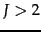classes. The method to use depends on whether the classes are mutually exclusive or not.

Classification for classes that are not mutually exclusive is called any-of , multilabel , or multivalue classification . In this case, a document can belong to several classes simultaneously, or to a single class, or to none of the classes. A decision on one class leaves all options open for the others. It is sometimes said that the classes are independent of each other, but this is misleading since the classes are rarely statistically independent in the sense defined on page 13.5.2 . In terms of the formal definition of the classification problem in Equation 112 (page 112 ), we learn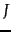different classifiers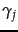in any-of classification, each returning either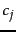or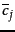: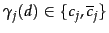.

Solving an any-of classification task with linear classifiers is straightforward:

1. Build a classifier for each class, where the training set consists of the set of documents in the class (positive labels) and its complement (negative labels).
2. Given the test document, apply each classifier separately. The decision of one classifier has no influence on the decisions of the other classifiers.

The second type of classification with more than two classes is one-of classification . Here, the classes are mutually exclusive. Each document must belong to exactly one of the classes. One-of classification is also called multinomial , polytomous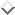, multiclass , or single-label classification . Formally, there is a single classification function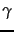in one-of classification whose range is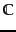, i.e.,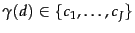. kNN is a (nonlinear) one-of classifier.

True one-of problems are less common in text classification than any-of problems. With classes like UK, China, poultry, or coffee, a document can be relevant to many topics simultaneously - as when the prime minister of the UK visits China to talk about the coffee and poultry trade.

Nevertheless, we will often make a one-of assumption, as we did in Figure 14.1 , even if classes are not really mutually exclusive. For the classification problem of identifying the language of a document, the one-of assumption is a good approximation as most text is written in only one language. In such cases, imposing a one-of constraint can increase the classifier's effectiveness because errors that are due to the fact that the any-of classifiers assigned a document to either no class or more than one class are eliminated.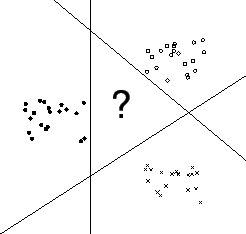hyperplanes do not divide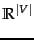intodistinct regions as illustrated in Figure 14.12 . Thus, we must use a combination method when using two-class linear classifiers for one-of classification. The simplest method is to rank classes and then select the top-ranked class. Geometrically, the ranking can be with respect to the distances from thelinear separators. Documents close to a class's separator are more likely to be misclassified, so the greater the distance from the separator, the more plausible it is that a positive classification decision is correct. Alternatively, we can use a direct measure of confidence to rank classes, e.g., probability of class membership. We can state this algorithm for one-of classification with linear classifiers as follows:

1. Build a classifier for each class, where the training set consists of the set of documents in the class (positive labels) and its complement (negative labels).
2. Given the test document, apply each classifier separately.
3. Assign the document to the class with
• the maximum score,
• the maximum confidence value,
• or the maximum probability.

 assigned class money-fx trade interest wheat corn grain true class money-fx 95 0 10 0 0 0 trade 1 1 90 0 1 0 interest 13 0 0 0 0 0 wheat 0 0 1 34 3 7 corn 1 0 2 13 26 5 grain 0 0 2 14 5 10
A confusion matrix for Reuters-21578.For example, 14 documents from grain were incorrectly assigned to wheat. Adapted from Picca et al. (2006).

An important tool for analyzing the performance of a classifier forclasses is the confusion matrix . The confusion matrix shows for each pair of classes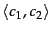, how many documents from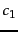were incorrectly assigned to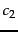. In Table 14.5 , the classifier manages to distinguish the three financial classes money-fx, trade, and interest from the three agricultural classes wheat, corn, and grain, but makes many errors within these two groups. The confusion matrix can help pinpoint opportunities for improving the accuracy of the system. For example, to address the second largest error in Table 14.5 (14 in the row grain), one could attempt to introduce features that distinguish wheat documents from grain documents.

Exercises.

• Create a training set of 300 documents, 100 each from three different languages (e.g., English, French, Spanish). Create a test set by the same procedure, but also add 100 documents from a fourth language. Train (i) a one-of classifier (ii) an any-of classifier on this training set and evaluate it on the test set. (iii) Are there any interesting differences in how the two classifiers behave on this task?Next: The bias-variance tradeoff Up: Vector space classification Previous: Linear versus nonlinear classifiers   Contents   Index